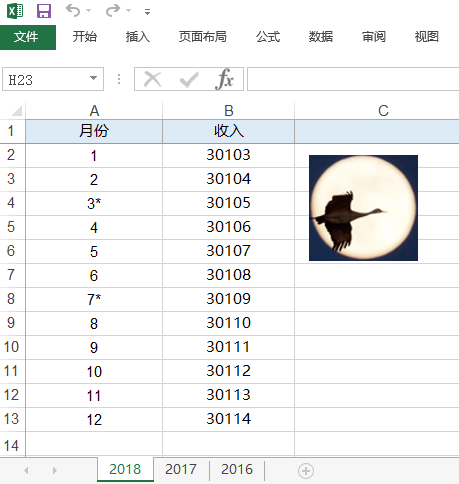# 读写Excel

Python开发，大家可以先根据我们网站自学。

## 读取Excel中的数据

``````pip install xlrd
``````xlrd 库里面的 open_workbook 函数打开Excel文件，并且返回一个 `Book对象` ，这个对象代表打开的 Excel 文件。

``````import xlrd

book = xlrd.open_workbook("income.xlsx")

print(f"包含表单数量 {book.nsheets}")
print(f"表单的名分别为: {book.sheet_names()}")
``````

``````# 表单索引从0开始，获取第一个表单对象
book.sheet_by_index(0)

# 获取名为2018的表单对象
book.sheet_by_name('2018')

# 获取所有的表单对象，放入一个列表返回
book.sheets()
``````

``````表单行数（nrows）

``````

``````import xlrd

book = xlrd.open_workbook("income.xlsx")

sheet = book.sheet_by_index(0)
print(f"表单名：{sheet.name} ")
print(f"表单索引：{sheet.number}")
print(f"表单行数：{sheet.nrows}")
print(f"表单列数：{sheet.ncols}")
``````

``````import xlrd

book = xlrd.open_workbook("income.xlsx")

sheet = book.sheet_by_index(0)

# 行号、列号都是从0开始计算
print(f"单元格A1内容是: {sheet.cell_value(rowx=0, colx=0)}")
``````

``````单元格A1内容是: 月份
``````

``````import xlrd

book = xlrd.open_workbook("income.xlsx")

sheet = book.sheet_by_index(0)

# 行号、列号都是从0开始计算
print(f"第一行内容是: {sheet.row_values(rowx=0)}")
``````

``````第一行内容是: ['月份', '收入']
``````

``````import xlrd

book = xlrd.open_workbook("income.xlsx")

sheet = book.sheet_by_index(0)

# 行号、列号都是从0开始计算
print(f"第一列内容是: {sheet.col_values(colx=0)}")
``````

``````第一列内容是: ['月份', 1.0, 2.0, 3.0, 4.0, 5.0, 6.0, 7.0, 8.0, 9.0, 10.0, 11.0, 12.0]
``````

``````import xlrd

book = xlrd.open_workbook("income.xlsx")

sheet = book.sheet_by_name('2017')

# 收入在第2列
incomes = sheet.col_values(colx=1,start_rowx=1)

print(f"2017年收入为: {sum(incomes)}")
``````

``````import xlrd

book = xlrd.open_workbook("income.xlsx")

sheet = book.sheet_by_name('2017')

# 收入在第2列
incomes = sheet.col_values(colx=1,start_rowx=1)

print(f"2017年账面收入为: {int(sum(incomes))}")

# 去掉包含星号的月份收入
toSubstract = 0
# 月份在第1列
monthes = sheet.col_values(colx=0)

for row,month in enumerate(monthes):
if type(month) is str and month.endswith('*'):
income = sheet.cell_value(row,1)
print(month,income)
toSubstract += income

print(f"2017年真实收入为: {int(sum(incomes)- toSubstract)}")
``````

``````import xlrd

book = xlrd.open_workbook("income.xlsx")

# 得到所有sheet对象
sheets = book.sheets()

incomeOf3years = 0
for sheet in sheets:
# 收入在第2列
incomes = sheet.col_values(colx=1,start_rowx=1)
# 去掉包含星号的月份收入
toSubstract = 0
# 月份在第1列
monthes = sheet.col_values(colx=0)

for row,month in enumerate(monthes):
if type(month) is str and month.endswith('*'):
income = sheet.cell_value(row,1)
print(month,income)
toSubstract += income

actualIncome = int(sum(incomes)- toSubstract)
print(f"{sheet.name}年真实收入为: {actualIncome}")
incomeOf3years += actualIncome

print(f'全部收入为{incomeOf3years}')
``````

## 新建Excel，写入数据

`xlrd` 只能读取Excel内容，如果你要 `创建` 一个新的Excel并 `写入` 数据，可以使用 `openpyxl` 库。

`openpyxl` 库既可以读文件、也可以写文件、也可以修改文件。

``````import openpyxl

# 创建一个Excel workbook 对象
book = openpyxl.Workbook()

# 创建时，会自动产生一个sheet，通过active获取
sh = book.active

# 修改当前 sheet 标题为 工资表
sh.title = '工资表'

# 保存文件
book.save('信息.xlsx')

# 增加一个名为 '年龄表' 的sheet，放在最后
sh1 = book.create_sheet('年龄表-最后')

# 增加一个 sheet，放在最前
sh2 = book.create_sheet('年龄表-最前',0)

# 增加一个 sheet，指定为第2个表单
sh3 = book.create_sheet('年龄表2',1)

# 根据名称获取某个sheet对象
sh = book['工资表']

# 给第一个单元格写入内容
sh['A1'] = '你好'

# 获取某个单元格内容
print(sh['A1'].value)

# 根据行号列号， 给第一个单元格写入内容，
# 注意和 xlrd 不同，是从 1 开始
sh.cell(2,2).value = '白月黑羽'

# 根据行号列号， 获取某个单元格内容
print(sh.cell(1, 1).value)

book.save('信息.xlsx')
``````

``````import openpyxl

name2Age = {
'张飞' :  38,
'赵云' :  27,
'许褚' :  36,
'典韦' :  38,
'关羽' :  39,
'黄忠' :  49,
'徐晃' :  43,
'马超' :  23,
}

# 创建一个Excel workbook 对象
book = openpyxl.Workbook()

# 创建时，会自动产生一个sheet，通过active获取
sh = book.active

sh.title = '年龄表'

# 写标题栏
sh['A1'] =  '姓名'
sh['B1'] =  '年龄'

# 写入内容
row = 2

for name,age in name2Age.items():
sh.cell(row, 1).value = name
sh.cell(row, 2).value = age
row += 1

# 保存文件
book.save('信息.xlsx')
``````

## 修改Excel中的数据

### 修改单元格内容

``````import openpyxl

# 加载 excel 文件

# 得到sheet对象
sheet = wb['2017']

sheet['A1'] = '修改一下'

## 指定不同的文件名，可以另存为别的文件
wb.save('income-1.xlsx')
``````

### 插入行、插入列

sheet 对象的 `insert_rows``insert_cols` 方法，分别用来插入 `行``列`， 比如

``````import openpyxl

sheet = wb['2018']

# 在第2行的位置插入1行
sheet.insert_rows(2)

# 在第3行的位置插入3行
sheet.insert_rows(3,3)

# 在第2列的位置插入1列
sheet.insert_cols(2)

# 在第2列的位置插入3列
sheet.insert_cols(2,3)

## 指定不同的文件名，可以另存为别的文件
wb.save('income-1.xlsx')
``````

### 删除行、删除列

sheet 对象的 `delete_rows``delete_cols` 方法，分别用来插入 `行``列`， 比如

``````
import openpyxl

sheet = wb['2018']

# 在第2行的位置删除1行
sheet.delete_rows(2)

# 在第3行的位置删除3行
sheet.delete_rows(3,3)

# 在第2列的位置删除1列
sheet.delete_cols(2)

# 在第3列的位置删除3列
sheet.delete_cols(3,3)

## 指定不同的文件名，可以另存为别的文件
wb.save('income-1.xlsx')
``````

### 文字 颜色、字体、大小

``````import openpyxl
# 导入Font对象 和 colors 颜色常量
from openpyxl.styles import Font,colors

sheet = wb['2018']

# 指定单元格字体颜色，
sheet['A1'].font = Font(color=colors.RED, #使用预置的颜色常量
size=15,    # 设定文字大小
bold=True,  # 设定为粗体
italic=True # 设定为斜体
)

# 也可以使用RGB数字表示的颜色
sheet['B1'].font = Font(color="981818")

# 指定整行 字体风格， 这里指定的是第3行
font = Font(color="981818")
for y in range(1, 100): # 第 1 到 100 列
sheet.cell(row=3, column=y).font = font

# 指定整列 字体风格， 这里指定的是第2列
font = Font(bold=True)
for x in range(1, 100): # 第 1 到 100 行
sheet.cell(row=x, column=2).font = font

wb.save('income-1.xlsx')
``````

### 背景色

``````import openpyxl
# 导入Font对象 和 colors 颜色常量
from openpyxl.styles import PatternFill

sheet = wb['2018']

# 指定 某个单元格背景色
sheet['A1'].fill = PatternFill("solid", "E39191")

# 指定 整行 背景色， 这里指定的是第2行
fill = PatternFill("solid", "E39191")
for y in range(1, 100): # 第 1 到 100 列
sheet.cell(row=2, column=y).fill = fill

wb.save('income-1.xlsx')
``````

### 插入图片

``````import openpyxl
from openpyxl.drawing.image import Image